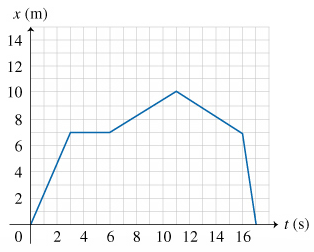# Find the object's speeds v1, v2, and v3 at times t1=2.0s, t2=4.0s, and t3=13s

Find the object’s speeds v1, v2, and v3 at times t1=2.0s, t2=4.0s, and t3=13s.